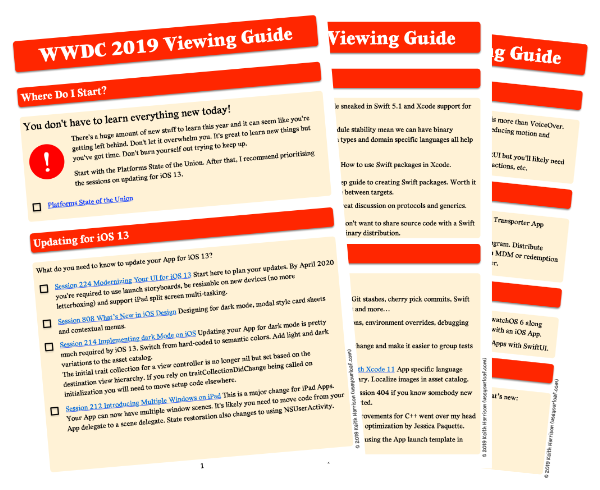# Updating Strings for Swift 3

I wrote a Swift String Cheat Sheet last year to help me remember how to use one of the more complex API’s in the Swift standard library. It has undergone some significant changes with Swift 3 making for some painful code migration. This is partly down to the new API naming guidelines but also because of a new model for the way collections, indices and ranges work.

These are my notes on what I needed to change to update the Swift playground for Swift 3.

### The Great Renaming

Applying the new API guidelines to the standard library has changed many of the `String` properties and methods. I will not mention every name change here as most are easily fixed by Xcode. Here are a couple of typical changes to give you the idea:

#### Initializing a String

The standard library replaces the `String` initializer `init(count:repeatedValue)` with `init(repeating:count)`. The repeatedValue is also now a `String` instead of a character which makes it more flexible:

``````// Swift 2
let h = String(count:3, repeatedValue:"0") // "000"

// Swift 3
let h = String(repeating:"01", count:3)    // 010101
``````

#### Converting to upper/lower case

The properties `uppercaseString` and `lowercaseString` are now functions and renamed to `uppercased()` and `lowercased()`:

``````let mixedCase = "AbcDef"

// Swift 2
// let upper = mixedCase.uppercaseString // "ABCDEF"
// let lower = mixedCase.lowercaseString // "abcdef"

// Swift 3
let upper = mixedCase.uppercased()       // "ABCDEF"
let lower = mixedCase.lowercased()       // "abcdef"
``````

I’ll cover some of the other name changes as we go.

### Using Index to Traverse a Collection

One of the biggest changes impacting `String` in Swift 3 is the new model for collections and indices. To recap you cannot directly access an element in a `String` instead you need to use an index on one of the collection views:

``````let country = "España"
country.characters       // characters
country.unicodeScalars   // Unicode scalar 21-bit codes
country.utf16            // UTF-16 encoding
country.utf8             // UTF-8 encoding
``````

Unchanged with Swift 3 are the `startIndex` and `endIndex` properties on each collection view:

``````let hello = "hello"
let helloStartIndex = hello.characters.startIndex // 0
``````

If you want the character view you can also omit the `characters` property:

``````let startIndex = hello.startIndex // 0
let endIndex = hello.endIndex     // 5
hello[startIndex]                 // "h"
``````

What has changed is the way you advance an index to traverse over a string view. The `successor()`, `predecessor()` and `advancedBy(n)` functions are all gone.

``````// Swift 2
hello[hello.startIndex]                // "h"
hello[hello.startIndex.successor()]    // "e"
hello[hello.endIndex.predecessor()]    // "o"
``````

In Swift 3 you now use `index(after:)` and `index(before:)` and `index(_:offsetBy:)` to achieve the same results:

``````// Swift 3
hello[hello.startIndex]                // "h"
hello[hello.index(after: startIndex)]  // "e"
hello[hello.index(before: endIndex)]   // "o"

hello[hello.index(startIndex, offsetBy: 1)]  // "e"
hello[hello.index(endIndex, offsetBy: -4)]   // "e"
``````

You can also limit the offset to avoid an error when you run off the end of the index. The function `index(_:offsetBy:limitedBy:)` returns an optional which will be `nil` if you go too far:

``````if let someIndex = hello.index(startIndex,
offsetBy: 4, limitedBy: endIndex) {
hello[someIndex] // "o"
}
``````

To find the index of the first matching element (in this case a character):

``````let matchedIndex = hello.characters.index(of: "l") // 2
let nomatchIndex = hello.characters.index(of: "A") // nil
``````

Finally the method to get the distance between two indices has been renamed:

``````// Swift 2
let distance = word1.startIndex.distanceTo(indexC)

// Swift 3
let distance = word1.distance(from: word1.startIndex, to: indexC)
``````

### Using Ranges

Ranges have changed in Swift 3. Suppose I have a start index (lowerBound) and end index (upperBound) on a characters view:

``````let fqdn = "useyourloaf.com"
let tldEndIndex = fqdn.endIndex
let tldStartIndex = fqdn.index(tldEndIndex, offsetBy: -3)
``````

The full initializer to create a range from the lower and upper bounds:

``````let range = Range(uncheckedBounds: (lower: tldStartIndex, upper: tldEndIndex))
fqdn[range]  // "com"
``````

The easier way to create a range is with the `..<` and `...` operators:

``````let endOfDomain = fqdn.index(fqdn.endIndex, offsetBy: -4)
let rangeOfDomain = fqdn.startIndex ..< endOfDomain
fqdn[rangeOfDomain] // useyourloaf
``````

Checking for and returning the range of a matching substring:

``````if let rangeOfTLD = fqdn.range(of: "com") {
let tld = fqdn[rangeOfTLD]                 // "com"
}
``````

### Playground

You can find the fully updated playground in its GitHub repository. I have also updated the original post.